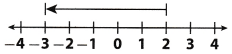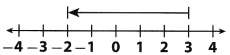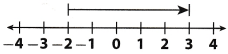Refer to our Texas Go Math Grade 6 Answer Key Pdf to score good marks in the exams. Test yourself by practicing the problems from Texas Go Math Grade 6 Module 5 Quiz Answer Key.

5.1 Adding Integers with the Same Sign

Question 1.
– 8 + (- 6) __________
First, find absolute values of given integers:
|- 8| = 8 and |- 6|= 6
Then, sum that absolute values
8 + 6 = 14
Final result would be 14 or – 14, depending on sign of given integers (both positive then +, both negative then -).
Given integers are negative so final result is – 14.

Result is – 14.
Find absolute values, sum them and add minus if both integers were negative.

Question 2.
– 4 + (- 7) __________
First, find absolute values of given integers:
|- 4| = 4 and |- 7|= 7
Then, sum that absolute values
4 + 7 = 11
Final result would be 11 or – 11, depending on sign of given integers (both positive then +, both negative then -).
Given integers are negative so final result is – 11.

Result is – 11.
Find absolute values, sum them and add minus if both integers were negative.

Question 3.
– 9 + (- 12) __________
First, find absolute values of given integers:
|- 9| = 9 and |- 12|= 12
Then, sum that absolute values
9 + 12 = 21
Final result would be 21 or – 21, depending on sign of given integers (both positive then +, both negative then -).
Given integers are negative so final result is – 21.

Result is – 21.
Find absolute values, sum them and add minus if both integers were negative.

5.2 Adding Integers with Different Signs

Question 4.
5 + (- 2) __________
Find absolute values and subtract lesser absolute value from greater:
| 5 | – |- 2| = 3
Final result would be 3 or -3, depending on signs of given integers.
Use the sign of number with greater absolute value to find the final result:
5 + (- 2) = 3

The sum is 3.
Find absolute values, subtract them and add minus if integer with greater absolute value was negative.

Question 5.
– 8 + 4 __________
Find absolute values and subtract lesser absolute value from greater:
| – 8 | – |4| = 4
Final result would be 4 or – 4, depending on signs of given integers.
Use the sign of number with greater absolute value to find the final result:
– 8 + 4 = – 4

The sum is – 4.
Find absolute values, subtract them and add minus if integer with greater absolute value was negative.

Question 6.
15 + (- 8) __________
Find absolute values and subtract lesser absolute value from greater:
| 15 | – |- 8| = 7
Final result would be 7 or – 7, depending on signs of given integers.
Use the sign of number with greater absolute value to find the final result:
15 + (- 8) = 7

The sum is 7.
Find absolute values, subtract them and add minus if integer with greater absolute value was negative.

5.3 Subtracting integers

Subtract.

Question 7.
2 – 9 __________
Subtracting 9 is equal to adding its additive inverse – 9 so you can find sum:
2 + (- 9)
To calculate sum, subtract lesser absolute value from greater:
|- 9| – |2| = 7
Result would be 7 or – 7, depending on signs of given integers.
Use the sign of integer with greater absolute value to find the sum:
2 + (- 9) = – 7
Since finding difference
2 – 9
is the same as finding sum
2 + (- 9)
the difference is – 7.

The difference is – 7
To subtract 9, add its additive inverse – 9 and find the sum using rules for adding integers.

Question 8.
– 3 – (- 4) __________
Subtracting – 4 is equal to adding its additive inverse 4 so you can find sum:
– 3 + 4
To calculate sum, subtract lesser absolute value from greater:
|4| – |- 3| = 1
Result would be 1 or – 1, depending on signs of given integers.
Use the sign of integer with greater absolute value to find the sum:
– 3 + 4 = 1
Since finding difference
– 3 – (- 4)
is the same as finding sum
– 3 + 4
the difference is 1.

The difference is 1.
To subtract – 4, add its additive inverse 4 and find the sum using rules for adding integers.

Question 9.
11 – (- 12) __________
Subtracting – 12 is equal to adding its additive inverse 12 so you can find sum:
11 + 12
The sum is:
11 + 12 = 23
Since finding difference
11 – (- 12)
is the same as finding sum
11 + 12
the difference is 23.
To subtract – 12, add its additive inverse 12 and find the sum using rules for adding integers.

5.4 Applying Addition and Subtraction of Integers

Question 10.
A bus makes a stop at 2:30, letting off 15 people and letting on 9. The bus makes another stop ten minutes later to let off 4 more people. How does the number of people on the bus after the second stop compare to the number of people on the bus before the 2:30 stop?
The expression that represents number of people on the bus after second stop is:
– 15 + 9 – 4
Subtracting 4 is equal to adding its additive inverse -4 so you can find sum:
– 15 + 9 + (- 4)
Use Commutative Property to simplify addition:
– 15 + (- 4) + 9
Use Associative Property and rules for adding integers to find the result:
– 19 + 9 = – 10
The number of people on the bus after second stop is Lesser than the number of people on the bus before first stop. There are 10 people less than before two stops.

Question 11.
Cate and Elena were playing a card game. The stack of cards in the middle had 24 cards in it to begin with. Cate added 8 cards to the stack. Elena then took 12 cards from the stack. Finally, Cate took 9 cards from the stack. How many cards were left in the stack?
Start with 24. Use positive integer to represent cards that are added to the stack and negative integer to represent cards that are taken from stack. The expression you get 15:
24 + 8 – 12 – 9
Subtracting 12 and 9 is equal to adding additive inverses – 12 and – 9 so you can find sum:
24 + 8 + (- 12) + (- 9)
Use Associative Property and rules for adding integers to find the result:
(24 + 8) + (- 12 + (- 9))
32 + (- 21) = 11
There were 11 cards left in the stack.
Use Associative Property and rules for adding integers to find the result

Essential Question

Question 12.
Write and solve a word problem that can be modeled by addition of two negative integers.
Sally withdrew $50 from her account on Tuesday and she withdrew$75 from her account on Friday. Find the total amount Sally withdrew on Tuesday and on Friday.
Since she withdrew money, write sum of negative integers to calculate the amount.
The sum ¡s:
– 50 + (- 75)
First, find absolute values of given integers:
|- 50| = 50 and |- 75| = 75
Then, sum that absolute values
50 + 75 = 125
Final result would be 125 or -125, depending on sign of given integers (both positive then +, both negative then -).
Given integers are negative so final result is – 125.
Sally withdrew $125 from her account. Sally withdrew$50 from her account on Tuesday and she withdrew $75 from her account on Friday. Find the total amount Sally withdrew on Tuesday and on Friday. She withdrew$125 from her account.

Texas Go Math Grade 6 Module 5 Mixed Review Texas Test Prep Answer Key

Selected Response

Question 1.
Which expression has the same value as – 3 + (- 5)?
(A) – 3 – (- 5)
(B) – 3 + 5
(C) – 5 + (- 3)
(D) – 5 – (- 3)
(C) – 5 + (- 3)

Explaination:
The expression is:
– 3 + (- 5)
Apply Commutative Property to that expression.
The expression you get is:
– 5 + (- 3)
To check, find both sums using rules for adding integers with the same sign:
– 3 + (- 5) = – 8
– 5 + (- 3) = – 8
Apply Commutative Property to the expression – 3 + (- 5) to get the expression with the same value.

Question 2.
A diver’s elevation is – 30 feet relative to sea level. She dives down 12 feet. What is her elevation after the dive?
(A) 12 feet
(B) 18 feet
(C) – 30 feet
(D) – 42 feet
(D) – 42 feet

Explaination:
Start with – 30 and add negative integer because she dives down. The expression you get iS:
– 30 + (- 12)
Find absolute values of given integers:
|- 30| = 30 and |- 12| = 12
Then, sum that absolute values
30 + 12 = 42
Final result would be 42 or – 42, depending on sign of given integers (both positive then +, both negative then -).
Given integers are negative so final result is – 42.
– 42 feet.
Find absolute values, sum them and add minus if both integers were negative.

Question 3.
Which number line models the expression – 3 + 5?
(A)(B)(C)(D)(B)Use number line to model that expression.
Start at – 3 and move 5 units to the right to represent adding 5.Question 4.
Which number can you add to 5 to get a sum of 0?
(A) – 10
(B) – 5
(C) 0
(D) 5
(B) – 5

Explaination:
To get a sum of 0, you need to add additive inverse of 5, which is – 5.
You get the expression:
5 + (- 5)
Its value is:
5 + (- 5) = 0

Question 5.
The temperature in the morning was – 3°F. The temperature dropped 11 degrees by night. What was the temperature at night?
(A) – 14°F
(B) – 8°T
(C) 8°F
(D) 14°F
(C) 8°F

Explaination:
The expression you get is:
– 3 + 11
To calculate sum, subtract lesser absolute value from greater:
|11| – |- 3| = 8
Result would be 8 or – 8, depending on signs of given integers.
Use the sign of integer with greater absolute value to find the sum:
– 3 + 11 = 8
8°F

Question 6.
Which of the following expressions has the greatest value?
(A) 3 – 7 + (- 10)
(B) 3 + 7 – (- 10)
(C) 3 – 7 – (- 10)
(D) 3 – 7 + (- 10)
(B) 3 + 7 – (- 10)

Explaination:
Use Commutative Property, Associative Property and rules for adding integers to calculate each sum and then compare results to find the greatest value.

(A) 3 – 7 + (- 10)
Subtracting 7 is equal to adding its additive inverse – 7 so you can find sum:
3 + (- 7) + (- 10)
Associative Property:
3 + ((- 7) + (- 10))
3 + (- 17) = – 14

(B) 3 + 7 – (- 10)
Subtracting – 10 is equal to adding its additive inverse 10 so you can find sum:
3 + 7 + 10
3 + 7 + 10 = 20

(C) 3 – 7 – (- 10)
Subtracting 7 and – 10 is equal to adding additive inverses – 7 and 10 so you can find sum:
3 + (- 7) + 10
Commutative Property:
3 + 10 + (- 7)
Associative Property:
13 + (- 7) = 6

(D) 3 + 7 + (- 10)
Associative Property:
10 + (- 10) = 0
The expression that has the greatest value is:
(B) 3 + 7 – (- 10)
Calculate each sum using rules for adding integers and compare results to find the greatest value.

Gridded Response

Question 7.
Mr. Renato had $150 in his bank account. He deposited$50. A couple of days later, he withdrew $20. A week later, he deposited$75. The next week he withdrew$100. What is Mr. Ranato’s balance after all the transactions?Answer: Add the integer if he deposited money and subtract integer if he withdrew ii The expression you get is: 150 + 50 – 20 + 75 – 100 Subtracting 20 and 100 is equal to adding additive inverses – 20 and – 100 so you can find sum: 150 + 50 + (- 20) + 75 + (- 100) Use Commutative Property and Associative Property to simplify addition: (150 + 50) + 75 + (- 20) + (- 100) Use Associative Property and rules for adding integers to find the result: 200 + 75 + ((- 20) + (- 100)) 275 + (- 120) = 155 His balance after alt the transactions ¡s$155.
Find the value of the expression 150 + 50 – 20 + 75 – 100 to calculate his balance.

Scroll to Top